# KSEEB SSLC Class 10 Maths Solutions Chapter 13 Statistics Ex 13.3

KSEEB SSLC Class 10 Maths Solutions Chapter 13 Statistics Ex 13.3 are part of KSEEB SSLC Class 10 Maths Solutions. Here we have given Karnataka SSLC Class 10 Maths Solutions Chapter 13 Statistics Exercise 13.3.

## Karnataka SSLC Class 10 Maths Solutions Chapter 13 Statistics Exercise 13.3

Question 1.
The following frequency distribution gives the monthly consumption of electricity of 68 consumers of a locality. Find the median, mean and mode of the data and compare them.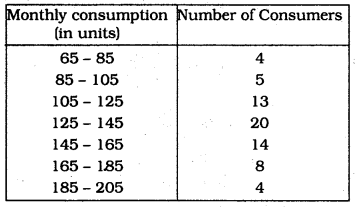Solution: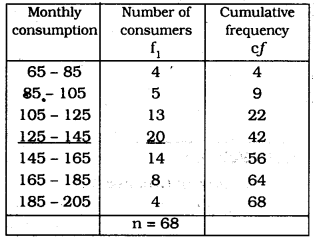(i) n = 68, ∴ $$\frac{n}{2}$$ = 34
The median is in class interval (125 – 145)
l = 125, n = 68, f = 20, cf = 22, h = 20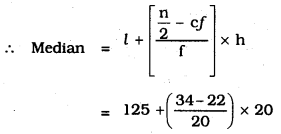= 125 + 12
∴ Median = 137 units.

(ii) To find out Mode: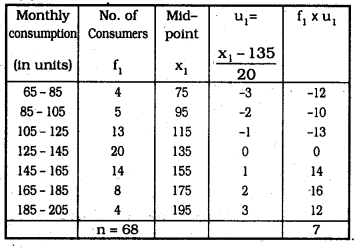Here, Mode is in class interval (125 – 145)
Maximum frequency, l = 125, f<sub>1</sub> = 20, f<sub>0</sub> = 13, f<sub>2/sub> = 14, h = 20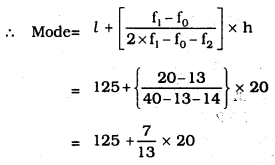= 125 + 10.76
= 135.76
∴ Mode = 135.76 units.

Question 2.
If the median of the distribution given below is 28.5, find the values of ‘x’ and ‘y’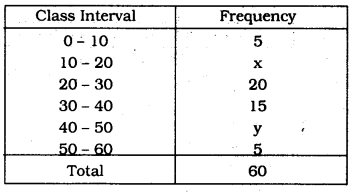Solution:
Median is 28.5
Class interval which has median is = (20 – 30)
l = 20, n = 60, f = 20, cf = 5 + a × h = 10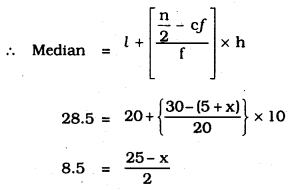17 = 25 – x
∴ x = 8
5 + x + 20 + 15 + y + 5 = 60
x + y – 45 = 60
x + y = 15
y = 15 – x
y = 15 – 8
∴ y = 7
∴ x = 8, y = 7

Question 3.
A life insurance agent found the following data for distribution of ages of 100 policy holders. Calculate the median age, if policies are given only to persons having age 18 years onwards but less than 60 year.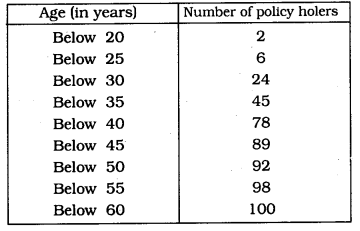Solution: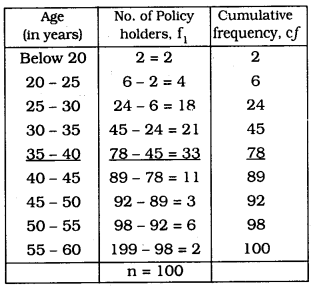n = 100 ∴ $$\frac{n}{2}$$ = 50
Median in which C.I. is (35 – 40)
l = 35, n = 100, f = 33, cf = 45, h = 5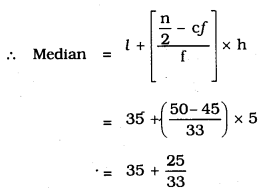= 35 + 0.76
∴ Median = 35.76 years

Question 4.
The lengths of 40 leaves of a plant are measured correct to the nearest millimetre, and the data obtained is represented in the following table: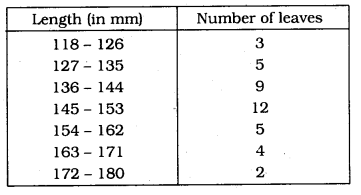Find the median length of the leaves.
(Hint: The data needs to be converted to continuous classes for finding the median, since the formula assumes continuous classes. The classes then change to 117.5 – 126.5, 126.5 – 135.5, 171.5 – 180.5)
Solution: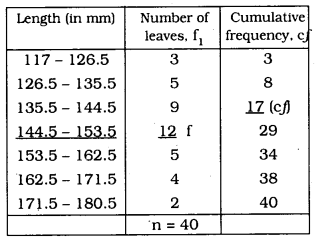n = 40 ∴ $$\frac{n}{2}$$ = 20
C.I. which has median is (144.5 – 153.5)
l = 144.5, n = 40, f= 12, cf = 17, h = 9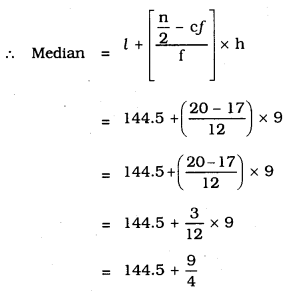= 144.5 + 2.25
∴ Median = 146.75 mm.

Question 5.
The following table gives the distribution of the lifetime of 400 neon lamps: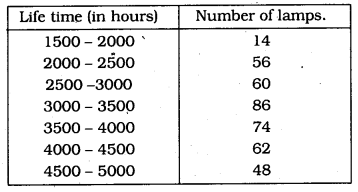Find the median life time of a lamp.
Solution: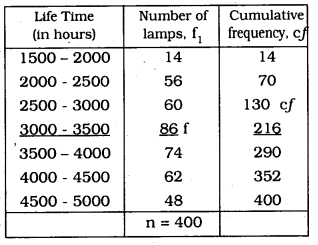n = 400 ∴ $$\frac{n}{2}=\frac{400}{2}=200$$
Class interval having median is = (3000 – 3500)
l = 3000, n = 400, f = 86, cf = 130, h = 500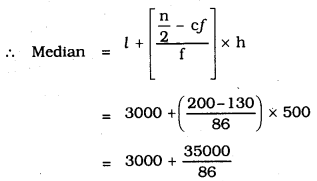= 3000 + 406.98
∴ Median = 3406.98 hours

Question 6.
100 surnames were randomly picked up from a local telephone directory and the frequency distribution of the number of letters in the English alphabets in the surnames was obtained as follows :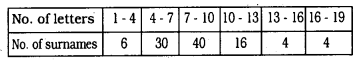Determine the median number of letters in the surnames. Find the mean number of letters in the surnames. Also, find the modal size of the surnames.
Solution: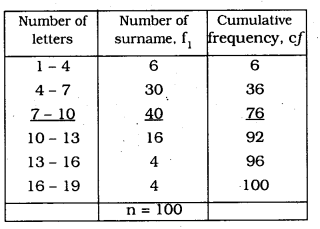(i) n = 100, ∴ $$\frac{n}{2}$$ = 50
Class interval having median is (7 – 10)
l = 7, n = 100, f = 40, cf= 36, h = 3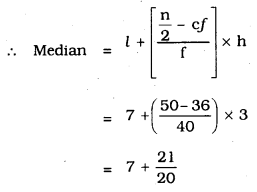= 7 + 1.05
∴ Median = 8.05 Letters

(ii) Clall interval which has mode is (7 – 10)
Maximum frequency, l = 7, f1 = 40, f0 = 30, f2 = 16, h = 3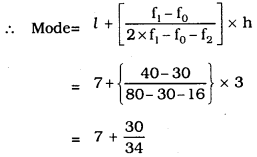= 7 + 0.88
∴ Mode = 7.88

(iii) Mean($$\overline{X}$$) : Step Deviation Method: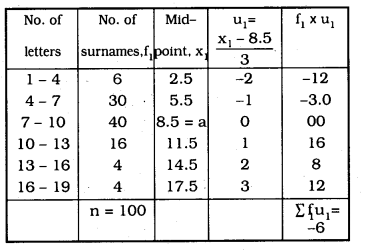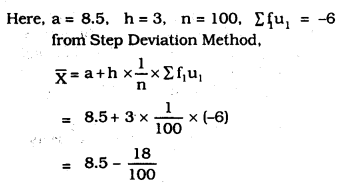= 8.5 – 0.18
= 8.32
∴ Mean = 8.32
∴ (i) Median = 8.05 letters
(ii) Mode = 7.88
(iii) Mean = 8.32.

Question 7.
The distribution below gives the weights of 30 students of a class. Find the median weight of the students.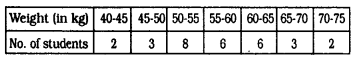Solution: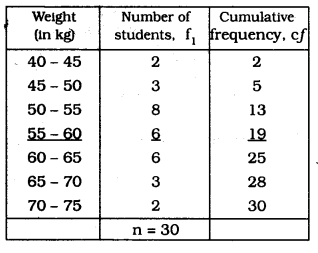(i) n = 30, ∴ $$\frac{\mathbf{n}}{2}$$ = 15
Class interval having median is (55 – 60)
l = 55, n = 30, f = 6, cf = 13, h = 5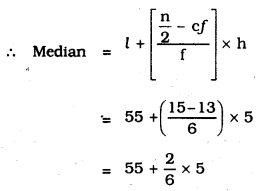= 55 + 1.67
∴ Median = 56.67 kg.

We hope the given KSEEB SSLC Class 10 Maths Solutions Chapter 13 Statistics Ex 13.3 will help you. If you have any query regarding Karnataka SSLC Class 10 Maths Solutions Chapter 13 Statistics Exercise 13.3, drop a comment below and we will get back to you at the earliest.